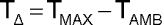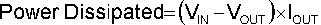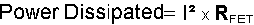Heatsink Requirements31 Aug 14 - Converted to JavaScript.

One thing that needs to be taken into account is ensuring that your regulator or FET doesn't overheat. Regulators are fitted with an internal over temperature cut-out, but you don't want the power dying when you trying to use the output.

The following table gives the thermal and power ratings for devices used on this website.

 Regulators xx = Output Voltage Thermal Co-efficients Maximum Permissible Junction Temperature (TMAX) Maximum Output Current (I) Maximum Power Dissipation (PMAX @ 25°C) Device Junction to Air (no heatsink) (θJA) Junction to Case (θJC) Case to Heatsink (θCHS) LM317T 50 °C/W 5 °C/W 0.5 °C/W 125 °C 1.5A 20W LM317LZ 180 °C/W N/A 160 °C/W 125 °C 100mA 625mW 78xx 65 °C/W 5 °C/W 0.5 °C/W 150 °C 1.5A 15W LT1084CP-xx N/A 0.65 °C/W 0.2 °C/W 125 °C 5A 45W LT1084CT-xx N/A 0.65 °C/W 0.2 °C/W 125 °C 5A 30W

 Regulator Heatsink Calculator Voltage Regulator Type = LM317T LM317LZ 78xx LT1084CP-xx LT1084CT-xx Input Voltage = V Output Voltage = V Output Current = A Ambient Temperature = °C Heatsink Required = °C/W

 FETs Thermal Co-efficients Maximum Permissible Junction Temperature (TMAX) Maximum Output Current (I) Maximum Power Dissipation (PMAX @ 25°C) Maximum Resistance when switched ON (RON) Device Junction to Air (no heatsink) (θJA) Junction to Case (θJC) IRF630 80 °C/W 1.67 °C/W 150 °C (100°C used) 9.0A @ 25°C 6.0A @ 100°C 75W @ 25°C 30W @ 100°C 0.4 Ω
 FET Heatsink Calculator Voltage Regulator Type = IRF630..... Current = A Ambient Temperature = °C Heatsink Required = °C/W

### Explanation:

The following shows the equations to calculate the need and necessary heatsink, with a worked example is at the bottom for a LM317T regulator. The maximum allowable temperature rise (TΔ) needs to be determined to ensure the regulator will not overheat can be determined using the following equation where TAMB = the Ambient Air Temperature around the device.For a voltage regulator,For a FET,The thermal coefficient required is given by:If the value of θ calculated by this equation is lower than the devices own heatsink effect (θJA) it will need an external heatsink. The heatsink required can be calculated using the following equation, where θCHS is the thermal coefficient between case and heatsink (normally taken as 0.5°C/W when using an insulating washer and thermal grease):Example:

• The input voltage to a LM317T regulator is 15V so that a stable 12V supply can be produced, but the output can also be at 5V.
• The maximum output current of 1.5A may be drawn so that is used for the calculations.
• The maximum temperature rise for the LM317T is worked out as TΔ = TMAX - TAMB = 125°C - 25°C = 100°C.
• The power dissipated will be (15v - 5v) x 1.5A = 15W
• The required thermal coefficient (θ) is therefore = 100°C / 15W = 6.67 °C/W
• This value is much lower than the devices own heatsink ability (50°C/W) so we will need an external heatsink.
• The required heatsink performance is θHS = 6.67°C/W - 5°C/W - 0.5°C/W = 1.17°C/W.
• This would be an extremely large heatsink, but it could be done especially is the case is metal and also forms part of the effective heatsink. Normally the difference between supply voltage and output is not so great and therefore a much small heatsink would be required (if at all).